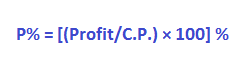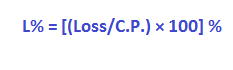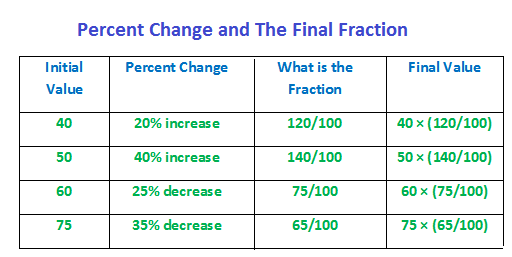## Profit and Loss

Definitions:

Cost Price (C.P.)

The price at which any article is purchased is its cost price.

Selling Price (S.P.)

The price at which an article is sold is its selling price.

Profit or Gain:

Profit is the difference between S.P. and C.P., if S.P. is greater than C.P

Loss:

Loss is the difference between C.P and S.P., if C.P. is greater than S.P

Profit percent (P %)

Profit percent is always computed over C.P. It is:Loss Percent (L %)

Loss percent is always computed over C.P., it is:Short-cut for Calculation:

Suppose the Cost Price of a book is 15. Let it be sold at 20% profit. What is the selling price?

S.P. = C.P. + Profit:

S.P. = 15 + (20/100) × 15 = 15 (1 + 20/100) = 15 × (120/100)

A 20% profit (increase) on 15 yields the product 15 × (120/100).

Writing this product enables speed in calculation.

Simplifying this product is easier compared to finding the amount of profit separately first and next adding it to the cost price.

Consider, therefore, more examples for further clarity:Solved Examples on the above concepts:

Example 1:

A book was purchased at 10. It was sold at 12. What is profit percent?

Solution:

C.P. = 10 and S.P. = 12. Profit = 12 ― 10 = 2.

Profit % is [(Profit/C.P.) × 100] %

(2/10) × 100 % = 20%

Example 2:

A boy purchased a pen at 5. He sold it his friend for 4. What is the loss he made in percentage?

Solution:

C.P. = 5 and S.P. = 4 and Loss = 5 ― 4 = 1.

Loss % is [(Loss/C.P.) × 100] %

(1/5) × 100 % = 20%

Example 3:

A book costs 75. At what price it should be sold to make a gain of 20%?

Solution:

From the short-cut for calculation discussed above:

S.P. = 75 × (120/100) = 90

Example 4:

A bag was sold at 90 with a loss of 25%. At what price it was purchased?

Solution:

Selling price (S.P.) 90 is 25% less than C.P.

From the above short cut, express selling price in terms of cost price as below:

90 = CP × (75/100) = ¾(C.P.)

Therefore, C.P. = 90 × (4/3) = 120

Marked Price, Selling Price and Discount:

The price marked on an article is its marked price (MP) or list price.

Discount is the rebate on marked price.

Discount is the is the difference between marked price and selling price (SP)

d = MP ― SP

Discount percent is always computed over MP. i.e.

d% = (d/MP) × 100%

Example 1:

A shirt marked at 40 was sold at 35. What is discount percent?

Solution:

Discount, d = 40 ― 35 = 5.

Applying the above formula d% = (d/MP) × 100%, the discount percentage is:

d% = (5/40) × 100% = 12.5%

Example 2:

A traveler’s bag was marked at 120. It was sold after being discounted by 25%. What was the selling price?

Solution:

Amount of discount is d = 25 % (120) = (25/100) × 120 = 30

Now, SP = MP – discount = 120 – 30 = 90

Short-cut:

We can as well apply the above short cut for calculation:

Since SP is 25% decrease (discount is decrease) on MP, we can write:

SP = (75/100) × 120 = 90

Example 3:

At what price should an object be marked to make a profit of 20% on its purchase price 80 after being discounted by 20%?

Solution:

Here, CP = 80.

SP = 20% increase (profit) on 80 (CP),

Therefore, SP = (120/100) × 80 = 96

Now, SP = 80% of MP, because of 20% discount on MP.

Therefore, SP = (80/100) × MP

i.e. 96 = (80/100) × MP

MP = 96 × (100/80) = 120